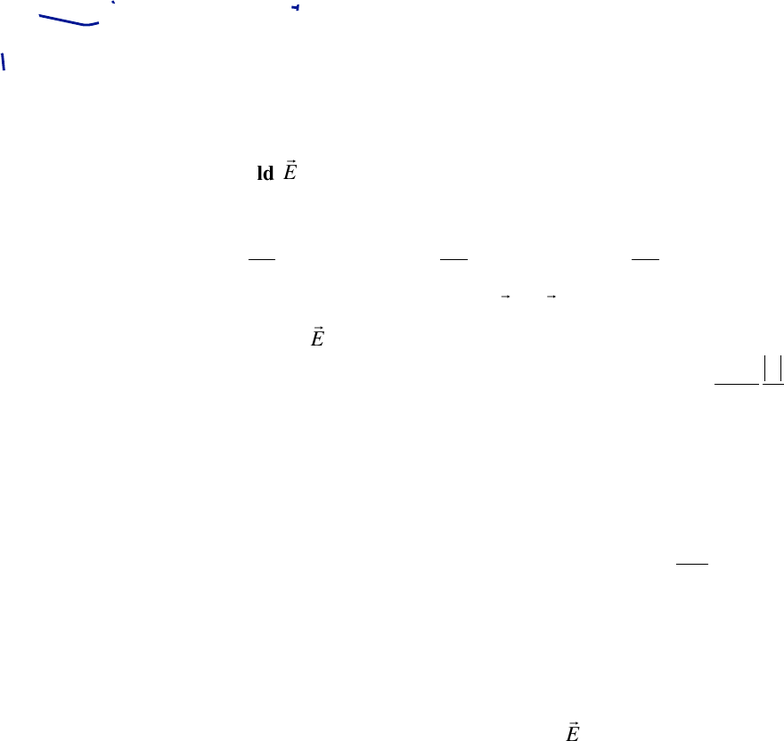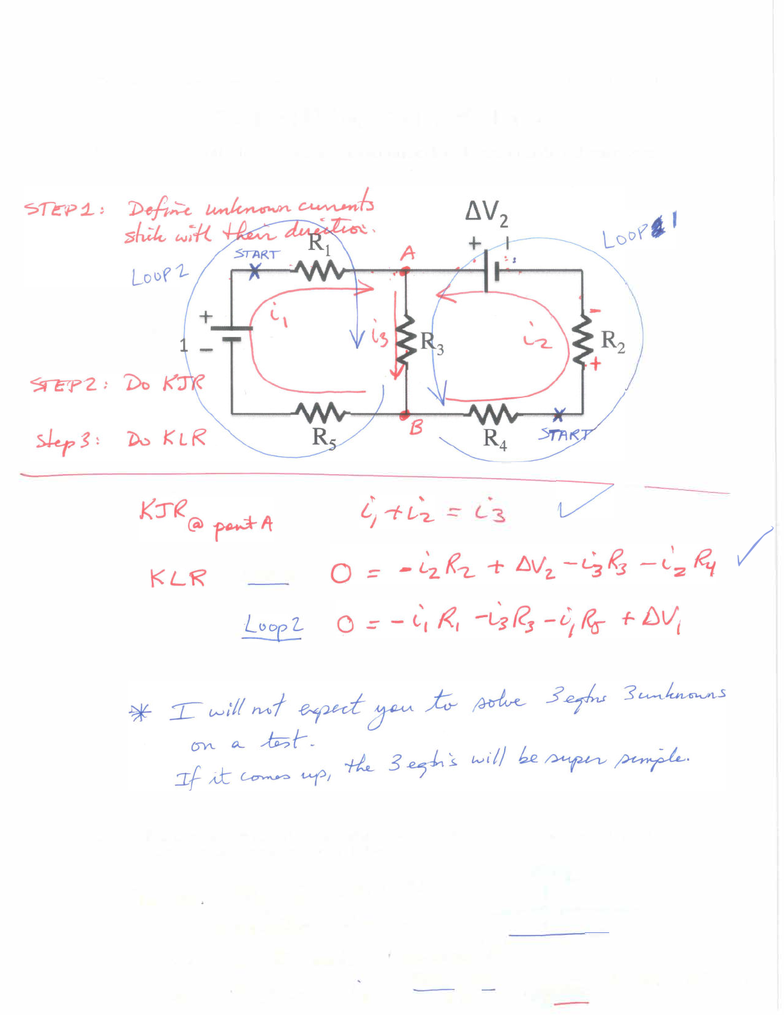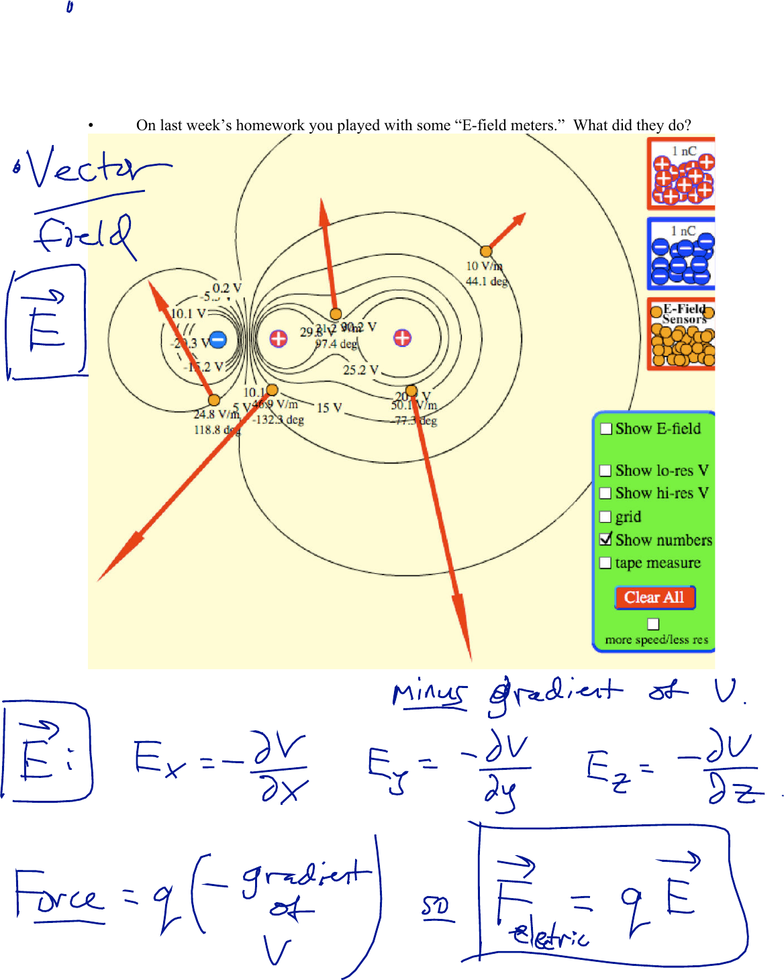Study Guides (400,000)
US (230,000)
Harvard (700)
(20)
Final

# Physical Sciences 3 Lecture 6: Lecture 3aExam

Department
Physical Sciences
Course Code
Physical Sciences 3
Professor
Roxanne Guenette
Study Guide
Final

This preview shows pages 1-3. to view the full 10 pages of the document.Physical Sciences 3: Lecture 3a
February 14, 2019
1
Electric Fields
The electric field
E
is a vector field, defined at every point in space. It can be
represented using field lines. It is equal to minus the gradient of the electric potential:
Ex=V
x
Ey=V
y
Ez=V
z
The electric force on a charge is given by:
F=q
E
. If q is negative, the force is in the
opposite direction of
E
.
The magnitude of the electric field from a single point charge is
E=1
4
πε
0
q
r
2
.
The field points away from a positive charge and towards a negative charge.
A pair of equal and opposite charges ±q separated by a distance d is a dipole, with dipole
moment p = qd. A dipole in an electric field will feel a torque that will tend to align the
dipole moment with the applied field.
The electric field in a parallel-plate capacitor is uniform:
E=q
ε
0A
An insulating material is called a dielectric. The electric field inside a dielectric is
reduced by a factor
κ
, called the dielectric constant. As a rule of thumb, in the presence
of a dielectric you should replace everywhere
ε
0
κε
0
. Water has
κ
= 80.
Learning objectives: After this lecture, you will be able to…
1. Define the electric field, calculate the electric field
E
from a given electric potential, and
sketch the field lines corresponding to a given electric field.
2. Use the electric field to calculate the electric force exerted on a charge.
3. Calculate, sketch, and explain the electric field created by a single point charge, or by an
electric dipole.
4. Calculate the torque exerted on an electric dipole in a uniform electric field, in terms of
the dipole moment.
5. Use the principle of superposition to find the electric field from several charges, or from a
continuous distribution of charge.
6. Calculate the electric field inside a parallel-plate capacitor with an air gap.
7. Determine the electric field in a conductor, in an empty cavity inside a conductor, or at
the surface of a conductor with a net charge.
8. Determine the electric field inside an insulator (a dielectric), assuming linear polarization
of the dielectric.
9. Explain how equations like Coulomb’s Law, or the capacitance of a parallel-plate
capacitor, are modified in the presence of a dielectric (like water).

Only pages 1-3 are available for preview. Some parts have been intentionally blurred.Only pages 1-3 are available for preview. Some parts have been intentionally blurred.Physical Sciences 3: Lecture 3a
February 14, 2019
2
The Electric Field
On last week’s homework you played with some “E-field meters.” What did they do?
We can define the electric field as:
Why is the electric field useful?